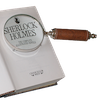#### You may also like### Double Digit### Number Detective

Follow the clues to find the mystery number.### Six Is the Sum

What do the digits in the number fifteen add up to? How many other numbers have digits with the same total but no zeros?

# Nice or Nasty

##### Age 7 to 14Challenge Level

Nice or Nasty - printable instructions sheet
Nice or Nasty - printable scoring sheet

Nice or Nasty printable sheet

Find a partner and a $1-6$ dice, or even a $0-9$ dice if you have one. You could use the dice in Dice and Spinners.

Each of you draw a set of four boxes like this:### Game $1$

Take turns to roll the dice and decide which of your four boxes to fill. Do this four times each until all your boxes are full. Read the four digits as a whole number.

#### Whoever has the larger four-digit number wins.

There are two possible scoring systems:

• A point for a win. The first person to reach $10$ wins the game
• Work out the difference between the two four-digit numbers after each round.
The winner keeps this score. First to $10000$ wins.

Now for some variations...

### Game $2$

Whoever makes the smaller four digit number wins. You'll probably want to change the scoring system.

### Game $3$

Set a target to aim for. Then throw the dice four times each and work out how far each of you is from the target number. Whoever is the closer wins.

There are two possible scoring systems:

• A point for a win. The first person to reach $10$ wins the game
• Work out the difference between the two four-digit numbers and the target number after each round. Keep a running total. First to $10000$ loses.

### Game $4$

This game introduces a decimal point. The decimal point will take up one of the cells so this time the dice only needs to be thrown three times by each player. Choose a target. The winner is the one closer to the target.

Two possible versions:

• each player decides in advance where they want to put the decimal point before taking turns to throw the dice
• each player throws the dice three times and then decides where to place the digits and the decimal point.

Again, two different scoring systems are possible.

### Game $5$

This is the nasty version!

Play any of the games above. This time you can choose to keep your number and put it in one of your cells, OR give it to your partner and tell them which cell to put it in. You might lose a friend this way! It's really important to take turns to start each round if this game is going to be fair.

This becomes even nastier when you play the games above with more than two people.

### Game $6$

A cooperative game rather than a competitive one - for three or more people.

Choose any of the games above. Decide in advance which of you will get the closest to the target, who will be second closest, third, fourth etc. Now work together to decide in whose cells the numbers should be placed, and where.